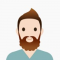## Queries to find distance between two nodes of a Binary tree – O(logn) method in C++Ayush Gupta

Updated on 09-Oct-2020 09:03:23

In this problem, we are given a binary tree and we are given Q queries. Our task is to create a program to solve Queries to find distance between two nodes of a Binary tree – O(logn) method in C++.Problem DescriptionIn each query, we are given two nodes of the ... Read More

## Queries to find maximum product pair in range with updates in C++Ayush Gupta

Updated on 09-Oct-2020 08:58:39

In this problem, we are given an array arr[] and Q queries. Each Query can be one of 2 types, 1st to find the maximum pair product in a given range [ Start - End ]. 2nd to update the ith index element with value. Our task is to create ... Read More

## Queries to find the last non-repeating character in the sub-string of a given string in C++Ayush Gupta

Updated on 09-Oct-2020 08:55:40

In this problem, we are given string str, and Q queries, each consisting of two integers. Our task is to create the program to solve Queries to find the last non-repeating character in the sub-string of a given string in C++.Problem DescriptionIn each query, we have two integers L and ... Read More

## Queries to find whether a number has exactly four distinct factors or not in C++Ayush Gupta

Updated on 09-Oct-2020 08:53:01

In this problem, we are given a Q number of queries, each having a number N. Our task is to create a program to solve the Queries to find whether a number has exactly four distinct factors or not in C++.Problem DescriptionTo solve each query, we need to find whether ... Read More

## Queries to add, remove and return the difference of maximum and minimum in C++Ayush Gupta

Updated on 09-Oct-2020 08:50:07

In this problem, we are given Q queries. These are of three types, they are −Query 1: Add number N to the list.Query 2: Remove number N to the list.Query 3: Return the difference of minimum and maximum element of the list.Our task is to create a program to solve ... Read More

## Program to find last digit of n’th Fibonnaci Number in C++Ayush Gupta

Updated on 09-Oct-2020 08:47:13

In this problem, we are given a number N. Our task is to create a Program to find last digit of Nth Fibonacci number in C++.Problem DescriptionWe need to find the last digit (i.e. LSB ) of the Nth Fibonacci number.Let’s take an example to understand the problem, Input: N ... Read More

## Program to find last two digits of 2^n in C++Ayush Gupta

Updated on 09-Oct-2020 07:40:41

In this problem, we are given a number N. Our task is to create a Program to find last two digits of 2^n in C++.Problem DescriptionTo find the last two digits. We will use only the product of the last two digits. And leave other things to make the calculation ... Read More

## Program to find LCM of two Fibonnaci Numbers in C++Ayush Gupta

Updated on 09-Oct-2020 07:34:02

In this problem, we are given two numbers N and M. Our task is to create a program to find LCM of two Fibonacci Numbers in C++.Problem Description − We will find the Nth and Mth Fibonacci number. And then we will find the LCM of their two numbers and ... Read More

## Program to find last two digits of Nth Fibonacci number in C++Ayush Gupta

Updated on 09-Oct-2020 07:22:00

In this problem, we are given a number N. Our task is to create a Program to find last two digits of Nth Fibonacci number in C++.Problem DescriptionWe need to find the last two digits (i.e. the two LSB’s ) of the Nth Fibonacci number. Let’s take an example to ... Read More

## Program to find Length of Bridge using Speed and Length of Train in C++Ayush Gupta

Updated on 09-Oct-2020 07:18:58

In this problem, we are given the length (L) and speed (S) of the train along with the time taken by it to pass the bridge. Our task is to create a program to find Length of Bridge using Speed and Length of Train in C++.Problem DescriptionWe need to find ... Read More

Previous 1 ... 3 4 5 6 7 ... 56 Next